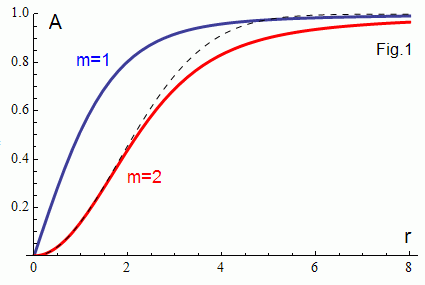Xo Yo delay fps
Use your fingers or mouse to control the model (hold shift key or use mouse wheel to zoom it). Canvas is matched to your browser window. -8 ≤ Xo,Yo ≤ 8.
You see m=2 vertex decay into two m=1 vorteces (in a while :)

# 2D vortex dynamicsNonlinear wave equation
t2u = Δu + (1 - |u|2)u
with complex u has stationary vortex solutions with the integer winding numbers m of the form
um(r) = Am(r) exp(imθ).
It is known  that the m = 1 vortices are stable and m ≥ 2 vortices are unstable. In this CPU based simulation you see m=2 vertex decay. Explicit scheme is used on a 250x250 grid. A simple approximation (the dashed curve) is used for the initial m=2 vertex. |u(x,y)| is plotted. Colors correspond to the u phase.

 "Quantized vortex stability and interaction in the nonlinear wave equation" Weizhu Bao, Rong Zengc, Yanzhi Zhang.

WebGL Demos     updated 27 September 2010